## Science Art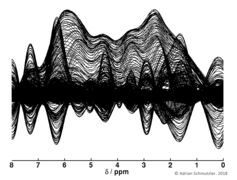### Black waves

Inherited from a former colleague. Don't know what it was supposed to be ...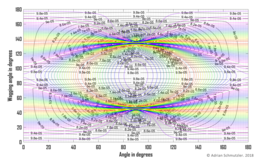### The eye

Quadrupolar tensor parameters based on jump angle variations.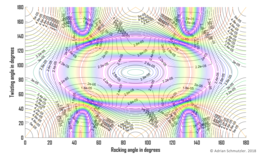### The big mountain

Quadrupolar tensor parameters based on jump angle variations.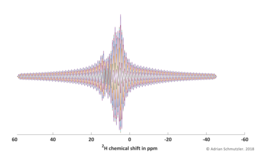Fourier transformation of a digitized free induction decay (FID) processed with the wrong group delay (introduces a first order phase shift of several 10000 Hz).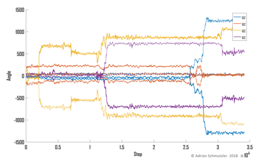### 1D Rorschach

Plot of Euler angles for a process where only the position of the intermediate y axis changes. As a result, the alpha and gamma angles are highly correlated. Hint: If you observe this, use Tait-Bryan angles (Wikipedia: Tait-Bryan angles).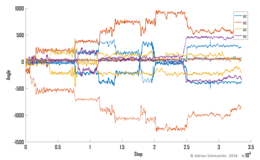### Mirror of waves

Plot of Euler angles for a process where only the position of the intermediate y axis changes. As a result, the alpha and gamma angles are highly correlated. Hint: If you observe this, use Tait-Bryan angles (Wikipedia: Tait-Bryan angles).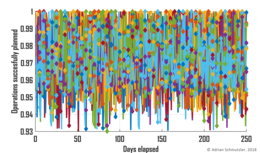### Carnival

Too much data ... Originating from a simulation for surgical workflow planning.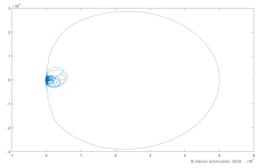### The knot

If you ever plot a spectrum, take only the real part. However, this is clearly a spectrum, since the real and imaginary parts are highly correlated in the Complex plane.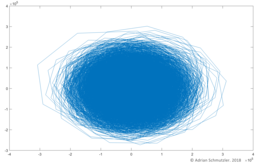### The egg

If you ever plot a spectrum, take only the real part. In this case, noise was plotted in the Complex plane.

# KONTAKT

eMail:

Telefon:

+49 (151) 566 1111 2

# Public Keys

Personal Public Key (PGP)

Server Public Key (SSH)